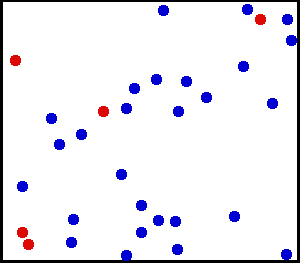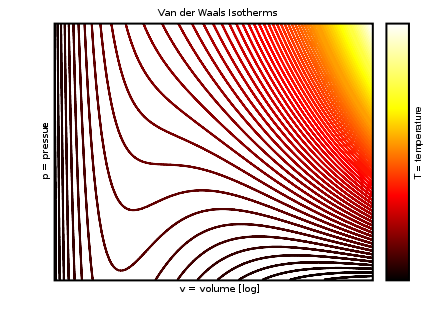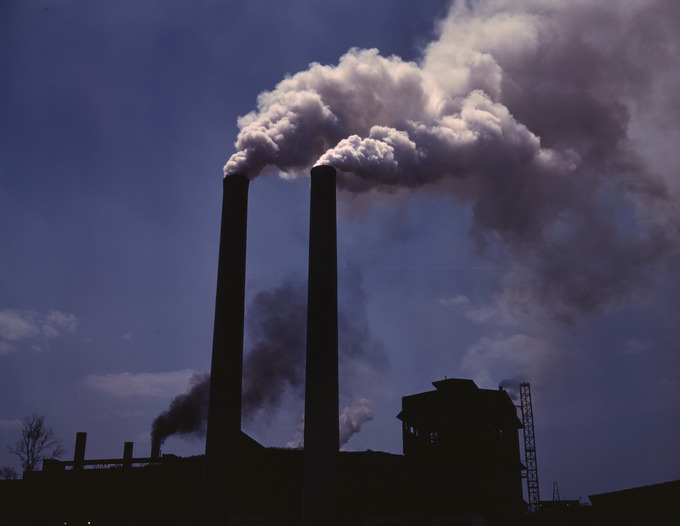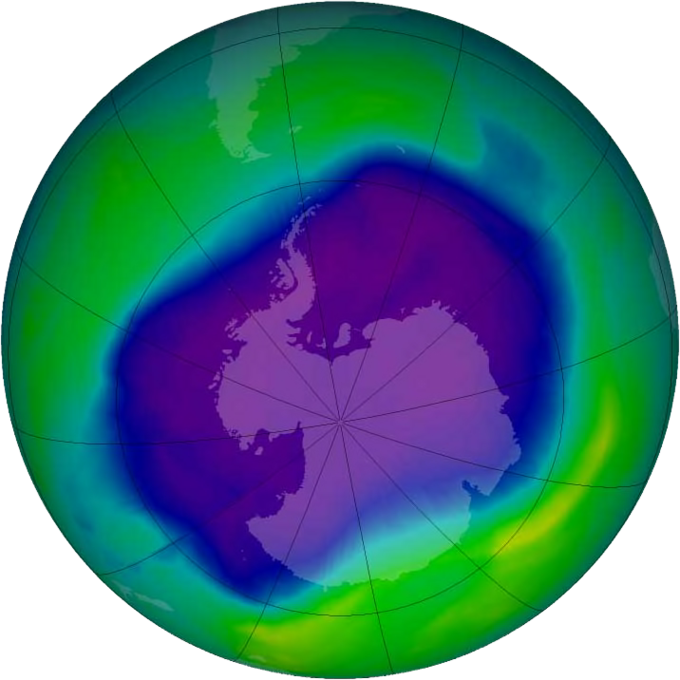The Effect of the Finite Volume

Real gases deviate from the ideal gas law due to the finite volume occupied by individual gas particles.

Learning Objectives

Demonstrate an understanding of the van der Waals equation for non-ideal gases.

Key Takeaways

Key Points

• The ideal gas law assumes that gases are composed of point masses that interact via completely elastic collisions.
• Real gases are made up of particles that occupy a non-zero volume known as the excluded volume.
• The van der Waals equation includes a volume-correction term that is specific to each gas; if a gas is behaving in an ideal manner, the correction term becomes negligible relative to the total volume.

Key Terms

• excluded volume: the volume occupied by non-ideal gas particles

The ideal gas law is commonly used to model the behavior of gas-phase reactions. Ideal gases are assumed to be composed of point masses whose interactions are restricted to perfectly elastic collisions; in other words, a gas particles’ volume is considered negligible compared to the container’s total volume.Gas particles: Ideal gases are assumed to be composed of point masses that interact via elastic collisions.

There are two notable situations in which the behavior of real gases deviates from that model:

1. At high pressures where the volume occupied by gas molecules does not approach zero
2. At low temperatures where the contribution of intermolecular forces becomes significant

Excluded Volume and the van der Waals Equation

The particles of a real gas do, in fact, occupy a finite, measurable volume. At high pressures, the deviation from ideal behavior occurs because the finite volume that the gas molecules occupy is significant compared to the total volume of the container. The van der Waals equation modifies the ideal gas law to correct for this excluded volume, and is written as follows:

$P(V - nb) = nRT$

The available volume is now represented as $V - nb$, where b is a constant that is specific to each gas. In this approximation, the gas molecules are considered hard spheres with a defined radius (r) that cannot overlap with the radius of a neighboring particle. The constant b is defined as:

$b = 4N_A \cdot \frac{4}{3} \pi \cdot r^{3}$

where NA is Avogadro’s number and r is the radius of the molecule.

It is important to note that this equation applies to ideal gases as well. It can be simplified because in an ideal situation, the value of b is so much smaller than V that it does not make a measurable difference in the calculation.Translational motion of helium: Under certain conditions, such as high pressure, real gases do not always behave according to the ideal model. Here, the size of helium atoms relative to their spacing is shown to scale under 1,950 atmospheres of pressure.

The Effect of Intermolecular Forces

At high pressures and low temperatures, intermolecular forces between gas particles can cause significant deviation from ideal behavior.

Learning Objectives

Discover how intermolecular forces result in gases deviating from ideal behavior.

Key Takeaways

Key Points

• Ideal gases are modeled as interacting through perfectly elastic collisions, implying that intermolecular interactions do not significantly contribute to the gas particles’ energetics.
• Real gas interactions, such as attractive and repulsive intermolecular forces, are more complex than perfectly elastic collisions; the significance of these contributions varies with the gases’ conditions.
• The van der Waals equation takes into account these intermolecular forces and offers an improved model for real gas behavior.

Key Terms

• intermolecular forces: attractive and repulsive forces between molecules

The Ideal Gas Law is a convenient approximation for predicting the behavior of gases at low pressures and high temperatures. This equation assumes that gas molecules interact with their neighbors solely through perfectly elastic collisions, and that particles exert no intermolecular forces upon each other.Elastic collisions between gas particles: Ideal gases are assumed to interact via perfectly elastic collisions in which no energy is lost.

Intermolecular forces describe the attraction and repulsion between particles. They include:

• Dipole -dipole forces
• Ion-dipole forces
• Dipole-induced dipole forces or Debye forces
• Instantaneous dipole-induced dipole forces or London dispersion forces.

The contribution of intermolecular forces creates deviations from ideal behavior at high pressures and low temperatures, and when the gas particles’ weight becomes significant.

Intermolecular Forces and the Limitations of the Ideal Gas Law

• At low temperatures, gas particles have less kinetic energy, and therefore move more slowly; at slower speeds, they are much more likely to interact (attracting or repelling one another) upon collision. The Ideal Gas Law does not account for these interactions.
• At high pressures, gas particles are forced into close proximity with one another, causing significant intermolecular interactions.
• When the weight of individual gas molecules becomes significant, London dispersion forces, or instantaneous dipole forces, tend to increase, because as molecular weight increases, the number of electrons within each gas molecule tends to increase as well. More electrons means that when two gas molecules collide or converge, the electron clouds around each nucleus can repel one another, thereby creating an “instantaneous dipole” (a separation of charge resulting in a partial positive and partial negative charge across the molecule). The dipoles can then induce further dipoles in neighboring molecules, and the unlike charges between molecules can attract one another. At high pressures and low temperatures, these attractive forces can become significant.

Correcting for the Intermolecular Forces of Real Gases

To correct for intermolecular forces between gas particles, J.D. van der Waals introduced a new term into the Ideal Gas Equation in 1873. By adding the term n2a/V2 to pressure, van der Waals corrected for the slight reduction in pressure due to the interaction between gas particles:

$P + \frac{an^2}{V^2}$

In the term above, a is a constant specific to each gas and V is the volume. van der Waals also corrected the volume term by subtracting out the excluded volume of the gas. The full van der Waals equation of state is written as:

$\left(P+\frac{an^2}{V^2}\right)\left(V-nb\right) = nRT$

where b is the excluded volume of the gas, R is the universal gas constant, and T is the absolute temperature.

Interactive: Charged and Neutral Atoms: Explore the role of charge in interatomic interactions.

Interactive: Seeing Intermolecular Attractions: Explore different types of attractions between molecules.

Van der Waals Equation

The van der Waals equation modifies the Ideal Gas Law to correct for the excluded volume of gas particles and intermolecular attractions.

Learning Objectives

Distinguish the van der Waals equation from the Ideal Gas Law.

Key Takeaways

Key Points

• The van der Waals equation is an equation of state that corrects for two properties of real gases: the excluded volume of gas particles and attractive forces between gas molecules.
• The van der Waals equation is frequently presented as: $\left(P + \frac{an^2}{V^2}\right)\left(V-nb\right) = nRT$.
• The constants a and b represent the magnitude of intermolecular attraction and excluded volume respectively, and are specific to a particular gas.

Key Terms

• ideal gas law: an equation describing the properties of an ideal gas, generally presented as PV = nRT
• van der waals equation: a relation between particles of a fluid that have a non-zero volume and a pairwise attractive inter-particle force

The Ideal Gas Law is based on the assumptions that gases are composed of point masses that undergo perfectly elastic collisions. However, real gases deviate from those assumptions at low temperatures or high pressures.  Imagine a container where the pressure is increased.  As the pressure increases, the volume of the container decreases.  The volume occupied by the gas particles is no longer negligible compared to the volume of the container and the volume of the gas particles needs to be taken into account.  At low temperatures, the gas particles have lower kinetic energy and do not move as fast.  The gas particles are affected by the intermolecular forces acting on them, which leads to inelastic collisions between them.  This leads to fewer collisions with the container and a lower pressure than what is expected from an ideal gas.

The van der Waals Equation and P-V Isotherms

Derived by Johannes Diderik van der Waals in 1873, the van der Waals equation modifies the Ideal Gas Law; it predicts the properties of real gases by describing particles of non-zero volume governed by pairwise attractive forces. This equation of state is presented as:

$\left(P + \frac{an^2}{V^2}\right)\left(V-nb\right) = nRT$

where P is the pressure, V is the volume, R is the universal gas constant, and T is the absolute temperature. Isotherm (plots of pressure versus volume at constant temperature) can be produced using the van der Waals model.van der Waals isotherms: This graph demonstrates the relationship between pressure, volume, and temperature based on the van der Waals model. It correctly predicts a mostly incompressible liquid phase, but the oscillations in the phase transition zone do not fit experimental data.

The constants a and b have positive values and are specific to each gas. The term involving the constant a corrects for intermolecular attraction. Attractive forces between molecules decrease the pressure of a real gas, slowing the molecules and reducing collisions with the walls.

• The higher the value of a, the greater the attraction between molecules and the more easily the gas will compress.
• The b term represents the excluded volume of the gas or the volume occupied by the gas particles.

Notice that the van der Waals equation becomes the Ideal Gas Law as these two correction terms approach zero.

The van der Waals model offers a reasonable approximation for real gases at moderately high pressures. Additional models have been subsequently introduced to more accurately predict the behavior of non-ideal gases.

Real Gases

Equations other than the Ideal Gas Law model the non-ideal behavior of real gases at high pressures and low temperatures.

Learning Objectives

Describe the five factors that lead to non-ideal behavior in gases and relate these to the two most common models for real gases

Key Takeaways

Key Points

• The Ideal Gas Law is a convenient approximation of most gas- phase reactions, but does not always sufficiently describe real gases near the condensation point, near the critical point, or at high pressures.
• Two common models for real gases are the van der Waals model and the Redlich-Kwong model.
• The deviation of a real gas from ideality can be quantified using the compressibility factor, Z.

Key Terms

• critical point: the temperature and pressure above which liquid and gas phases become indistinguishable; above the critical point, a substance exists as neither a liquid nor gas, but as a “supercritical fluid”
• isotherm: in thermodynamics, a curve on a P-V diagram representing the state of a gas at constant temperature (“iso-” means same and “-therm” refers to temperature)
• specific heat: the amount of heat necessary to raise one gram of a substance by one degree Celsius

Isotherms and the P-V Diagram

The Ideal Gas Law assumes that a gas is composed of randomly moving, non-interacting point particles. This law sufficiently approximates gas behavior in many calculations; real gases exhibit complex behaviors that deviate from the ideal model, however, as shown by the isotherms in the graph below. (Isotherms refer to the different curves on the graph, which represent a gas’ state at different pressure and volume conditions but at constant temperature; “Iso-” means same and “-therm” means temperature—hence isotherm.)Real-gas isotherms: According to the Ideal Gas Equation, PV=nRT, pressure and volume should have an inverse relationship. Notice that the higher isotherms on the graph, which represent the gas’ state at higher temperature, show the typical, concave decreasing curve of an inverse relationship. As temperature decreases, however, the isotherms on the lower portion of the graph significantly deviate from this ideal inverse relationship between P and V.

Limitations of the Ideal Model

For most applications, the ideal gas approximation is reasonably accurate; the ideal gas model tends to fail at lower temperatures and higher pressures, however, when intermolecular forces and the excluded volume of gas particles become significant. The model also fails for most heavy gases (including many refrigerants) and for gases with strong intermolecular forces (such as water vapor). At a certain point of combined low temperature and high pressure, real gases undergo a phase transition from the gaseous state into the liquid or solid state. The ideal gas model, however, does not describe or allow for phase transitions; these must be modeled by more complex equations of state.

Real-gas models must be used near the condensation point of gases (the temperature at which gases begin to form liquid droplets), near critical points, at very high pressures, and in other less common cases. Several different models mathematically describe real gases.

van der Waals Model

Real gases are often modeled by taking into account their molar weight and volume:

$\left(P + \frac{an^2}{V^2}\right)\left(V - nb\right)=nRT$

where:

• P = pressure
• T = temperature
• R = the ideal gas constant
• V = volume
• a is an empirically determined factor that corrects for the intermolecular forces between gas particles; it is specific for each gas
• b is an empirically determined factor that corrects for the excluded volume of gas particles; it is specific for each gas

Redlich-Kwong Model

The Redlich-Kwong equation is another two-parameter relation that models real gases. It is almost always more accurate than the van der Waals equation and frequently more accurate than some equations with more than two parameters. The equation is:

$RT = P(V_{m} - b) + a \cdot \frac{V_{m} - b}{V_{m} \cdot (V_{m} + b) \cdot T^{1/2}}$

Note that a and b here are defined differently than in the van der Waals equation.

Additional models that can be applied to non-ideal gases include the the Berthelot model, the Dieterici model, the Clausius model, the Virial model, the Peng-Robinson model, the Wohl model, the Beattie-Bridgeman model, and the Benedict-Webb-Rubin model. However, these systems are used less frequently than are the van der Waals and Redlich-Kwong models.

Compressibility Factor and Deviation from Ideality

Deviation from ideality can be quantified using the compressibility factor Z:

$Z = \frac{PV}{nRT}$

Note that for an ideal gas, PV=nRT, and Z will equal 1; under non-ideal conditions, however, Z deviates from unity. The graph below depicts how the compressibility factor varies with increasing pressure for a generalized graph. Note that the isotherms representing high temperatures deviate less from ideal behavior (Z remains close to 1 across the graph), while for isotherms representing low temperatures, Z deviates greatly from unity.Compressibility factor and pressure: At low temperatures, the compressibility factor for a generalized gas greatly deviates from unity, indicating non-ideal gas behavior; at high temperatures, however, the compressibility factor is much less affected by the increased pressure.

Air Pollution

Air pollution results from increasing levels of harmful molecules and particulates in the atmosphere.

Learning Objectives

Identify the four major effects of air pollution.

Key Takeaways

Key Points

• Air pollution results from the introduction of harmful compounds into the atmosphere.
• Pollutants are introduced from both natural and man-made sources.
• Major pollutants include particulate matter, ozone, sulfur dioxides, and nitrogen dioxide.

Key Terms

• acid rain: acidic precipitation, the result of sulfur dioxide and nitrogen oxides reacting with water in the atmosphere

Air pollution is the presence of harmful molecules or particulates, both natural and man-made, in the atmosphere.

Effects of Air Pollution

Increases in these atmospheric molecules and particles result in:

1. The greenhouse effect: an elevation in the Earth’s surface temperature due to the absorption of electromagnetic radiation by atmospheric gases.
2. Decreased stratospheric ozone: a depletion in ozone levels caused by the release of refrigerants, which produce free radicals that catalyze the decomposition of ozone.
3. Acid rain: acidic precipitation that occurs when the pollutants sulfur dioxide and nitrogen oxide react with water in the atmosphere.
4. Increased ozone concentrations at ground levels: an increase in surface ozone that contributes to smog.Air pollution: The output of industrial manufacturing processes is a major source of air pollution.

Major Pollutants and Their Effects

The main air pollutants produced by humans are:

1. Sulfur oxides (SOx)
2. Nitrogen oxides (NOx)
3. Carbon monoxide (CO)
4. Carbon dioxide (CO2)
5. Volatile organic compounds (VOCs)
6. Particulates
8. Metals
9. Chlorofluorocarbons (CFCs)
10. Ammonia (NH3)
11. Odors

Air pollutants are considered primary when the harmful particles are directly emitted into the atmosphere; secondary pollutants are products of reactions that occur following emission. Secondary pollutants include:

1. Smog
2. Ground level ozone (O3)
3. Peroxyacetyl nitrate

Air pollution is also a problem indoors, where poor health has been linked to pollutants like radon, VOCs, lead paint, combustion particulates, carbon monoxide, and asbestos. Air pollution contributes to poor health through respiratory conditions, heart disease, and lung cancer. Approximately 3.3 million deaths worldwide are attributed to indoor and outdoor air pollution, with children in developing countries at particular risk for illness or disease.

Ozone Depletion

Free radicals in the upper stratosphere act as catalysts for ozone decomposition, thereby depleting the ozone layer.

Learning Objectives

Sketch the ozone cycle.

Key Takeaways

Key Points

• Photochemical reactions in the stratosphere lead to the conversion of oxygen gas (O2) into atomic oxygen (O) and ozone (O3); these forward and reverse reactions are in very delicate balance.
• Free radicals, such as those from refrigerants, can catalyze the destruction of stratospheric ozone, resulting in large-scale depletion of ozone levels.
• Refrigerants such as CFCs, freons, and halons dissociate to produce free-radical forms of bromine and chlorine in the stratosphere.
• There is a gross misconception that CFC molecules are too heavy to reach the stratosphere and therefore do not contribute to ozone depletion; in fact, CFC molecules can behave as ideal gases and diffuse throughout Earth’s atmosphere, including the upper atmosphere.

Key Terms

• refrigerant: a substance used in a heat cycle that undergoes a phase change between gas and liquid to allow cooling, as in refrigerators and air conditioners
• photodissociation: molecular dissociation resulting from the absorption of a photon
• halocarbon: any compound derived from a hydrocarbon by replacing at least one hydrogen atom with a halogen; common examples include CFCs, or chlorofluorocarbons
• stratosphere: the region of the uppermost atmosphere where temperature increases with the altitude due to the ozone’s absorption of solar ultraviolet radiation; extends from the tropopause (10-15 kilometers) to approximately 50 kilometers, where it is succeeded by the mesosphere

Ozone’s Role in the Stratosphere

Ozone depletion describes two distinct but related phenomena observed since the late 1970s: (1) a steady decline of about 4 percent per decade in the total volume of ozone in Earth’s stratosphere (the ozone layer ); and (2) a much larger springtime decrease in stratospheric ozone over Earth’s polar regions. The latter phenomenon is referred to as the ozone hole. Both types of ozone depletion have increased as emissions of halo-carbons have increased.Largest ozone hole observed: In September, 2006, the average area of the ozone hole, displayed here in purple, was 10.6 million square miles.

The most important process in hole formation is the catalytic destruction of ozone by atomic halogens. The main source of these halogen atoms in the stratosphere is photodissociation of man-made halocarbon refrigerants; examples include CFCs (chlorofluorocarbons), freons, and halons. These compounds are emitted on Earth’s surface and move into the stratosphere.

CFCs and other contributing substances are referred to as ozone-depleting substances (ODS). Since the ozone layer prevents most harmful wavelengths (280-315 nm) of ultraviolet (UV) light from passing through Earth’s atmosphere, observed and projected decreases in ozone have generated worldwide concern. The Montreal Protocol bans the production of ozone-depleting chemicals such as carbon tetrachloride and trichloroethane.

Because the ozone layer acts as a protectant, blocking most UVB wavelengths of UV light from piercing Earth’s atmosphere, ozone depletion may result in a variety of biological consequences; these include increased cases of skin cancer, cataracts, and plant damage, as well as reduced plankton populations in the ocean’s photic zone. The largest ozone hole was observed in September, 2006.

Ozone Cycle

The ozone-oxygen cycle involves three forms of oxygen:

• Atomic oxygen (O)
• Diatomic oxygen, or oxygen gas (O2)
• Ozone (O3)

In the stratosphere, absorption of ultraviolet photons results in the photodissociation (breaking apart) of oxygen molecules. These atomic oxygen (O) radicals react with oxygen gas (O2) to produce ozone (O3); ozone’s absorption of ultraviolet light can then cause oxygen gas to re-form. The balanced equation for this reaction is:

$\text{O}+\text{O}_3\leftrightarrow{2}\text{O}_2$

Ozone Destruction

The balance of ozone production and degradation can be disturbed by the presence of competing free radicals, most notably the hydroxyl radical (OH·), nitric oxide radical (NO·), atomic chlorine radical (Cl·), and atomic bromine radical (Br·). The reaction of these free radicals with ozone disrupts the ozone-oxygen cycle, leading to the destruction of stratospheric ozone and the depletion of the ozone layer. The atomic chlorine and bromine radicals are found in certain stable organic compounds, especially CFCs, which can make their way to the stratosphere because of their low reactivity. Once in the stratosphere, ultraviolet light liberates the Cl and Br atoms from their parent compounds:

CFCl3 + electromagnetic radiation $\rightarrow$ CFCl2 + Cl

The Cl and Br atoms can then destroy ozone molecules through a variety of catalytic cycles. In the simplest example of such a cycle, a chlorine atom reacts with an ozone molecule, taking an oxygen atom (forming ClO, chlorine monoxide) and leaving a normal oxygen molecule (O2). The chlorine monoxide can then react with a second molecule of ozone (O3) to yield another chlorine atom and two molecules of oxygen. The chemical equations for these gas-phase reactions are:

$\text{Cl}^- +\text{O}_3\rightarrow\text{ClO}+\text{O}_2$

$\text{ClO}^- +\text{O}_3\rightarrow\text{Cl}+2\text{O}_2$

Thus, the atomic chlorine radical regenerates; a single chlorine can keep destroying ozone (acting as a catalyst ) for up to two years. The overall effect is a decrease in the amount of ozone, though null cycles can decrease the rate of these processes. More complicated mechanisms that lead to ozone destruction in the lower stratosphere have also been been discovered.

Diffusion of CFCs

A common misconception is that because CFC molecules are heavier than air (both nitrogen and oxygen), they cannot reach the stratosphere in significant amounts and therefore do not contribute significantly to ozone depletion. Atmospheric gases are not sorted by weight, however; wind forces can fully mix the gases in the atmosphere, which readily diffuse into the stratosphere.